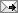### Question:: lagrangian

September 02, 2012
 Asked by: arshiya Title*: lagrangian Question*: what is basically the lagrangian function represents?
posted by S @ 12:21 AM• At October 8, 2012 at 10:15 AM,Prof. Balakrishnan said…

Lagrangian function L = T-V . where T and V are the kinetic energy and V is the potential energy of a system. Given a problem find an equation for T and V. and get the difference. In simple problems these can be obtained in terms of x,y z coordinates or in terms of r theta and phi. Google for Lagrangian of different systems or search for Lagrangian homework with solutions you will get the L for many systems. rbk

• At October 28, 2012 at 4:40 PM,Anonymous said…

The Lagarangian has no physical significance but it is the mathematical function devised to obtain the equations of motion

• At November 21, 2012 at 2:03 PM,Jitendra Kr. Soni said…

Lagrangian function is basically represented by L and its nothing but difference between the kinetic energy and potential energy.
L = T - V , where T is kinetic and V is potential energy.

• At November 22, 2012 at 1:57 PM,sanjeev said…

a function that describes the state of a dynamic system in terms of position coordinates and their time derivatives and that is equal to the difference between the potential energy and kinetic energy

• At March 13, 2013 at 10:52 AM,Anonymous said…

L=T-V